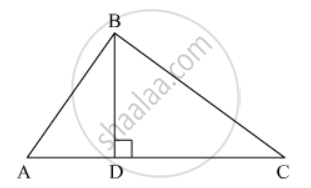# Prove That, in a Right Triangle, the Square on the Hypotenuse is Equal to the Sum of the Squares on the Other Two Sides. - Mathematics

Prove that, in a right triangle, the square on the hypotenuse is equal to the sum of the squares on the other two sides.

#### SolutionGiven: A right ΔABC right angled at B

To prove : AC2 = AB2 + BC2

∴ ΔADB ∼ ΔABC  (by AA similarly criterion)

=> (AD)/(AB) = (AB)/(AC)

⇒ AD × AC = AB2    ...... (1)

Now In ΔBDC and ΔABC

∠BDC = ∠ABC = 90°

∠BCD = ∠BCA  (common)

∴ ΔBDC ∼ ΔABC  (by AA similarly criterion)

=> (CD)/(BC) = (BC)/(AC)

⇒ CD × AC = BC2    ........ (2)

Adding (1) and (2) we get

AB2 + BC2 = AD × AC + CD × AC

= AC × AC = AC2

∴ AC2 = AB2 + BC2

Hence Proved.

Concept: Similarity of Triangles
Is there an error in this question or solution?